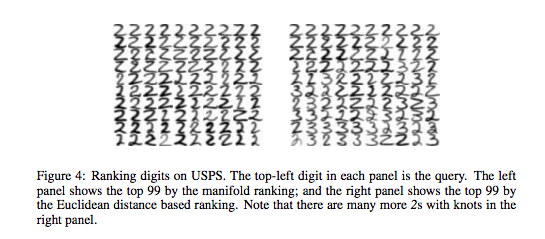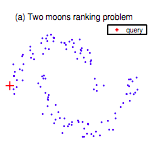# PageRank meets vectorial representations – "Ranking on Data Manifolds"

I came across this paper when following up on ideas I had when reading about TextRank for summarising documents. It is short, well written and very interesting, and was authored by Zhou, Weston, Gretton, Bousquet and Schölkopf (all then at the Max Planck Institute for Biological Cybernetics, Tübingen) in 2004. (PDF).

The authors consider the problem of ranking objects by relevancy to one or more query objects in the case where the objects have a vectorial representation. This is done using the PageRank algorithm on a graph in which the vertices represent the objects and the edges weights are computed using e.g. an RBF kernel (or normalised dot-product, if the vectors are non-negative).

One advantage of this approach is that it generalises naturally to multiple query vectors. The query vectors are simply treated as a complete list of possible (re)starting points for the PageRank random walk. This contrasts with the typical PageRank case, where all pages are possible starting points. Note that this list of starting points need not be binary — it can rather be a probability distribution over the objects representing user preference.

The PageRank approach is shown to perform much better than Euclidean nearest neighbours search on real world data sets (MNIST digits and newsgroup posts are considered). In both cases, the datasets have labels. The query data points are chosen from a single class, and the ranking problem is treated as one of binary classification, i.e. finding the distinguishing the objects of the same class from those of all other classes. The ranking is used to calculate a ROC curve, and the area under this curve is used as a performance measure.

The evaluation considers the case of multiple query vectors, as well. The Euclidean nearest neighbours, in the multiple query vector case, are aggregated by taking the minimal distance to a query vector (i.e. “disjunctively”).

The PageRank method is visually contrasted with the Euclidean nearest neighbours case using the MNIST data set. Below are the 99 best ranked results in PageRank case (left) and the Euclidean case (right) (99 = 10 x 10 – 1 query). Not only does the left panel contain no threes, but the twos are more homogeneous.The graph that the authors construct is not complete. Rather, edges are added, beginning with those most heavily weighted (but excluding self-loops) until the graph is connected. For example, in the case of the two sickle moons:Hyperparameters
An RBF kernel function is used in some cases to define the edge weighting between nodes (see step 2 of the algorithm). Note that the varianceof the RBF kernel needs to be fitted using cross-validation. This is no problem for labelled datasets like MNIST, but would be problematic for e.g. the sickle moons data.

It’s not a random walk
Note that, due to the “symmetric normalisation” that is applied to the affinity matrix(see step 3 of the algorithm), this is not a random walk — the columns of the normalisationdo not sum to 1, so the iterative procedure of step 4 will not map probability distributions to probability distributions. Given that we only want to rank the nodes, not derive a probability distribution over them, this is not necessarily a problem.

Why symmetric normalisation? Dengyong was kind to explain the motivation for this (via correspondence). This normalisation was used in order to avoid over-emphasising points in high density regions, and because it is the normalised graph Laplacian (I haven’t looked into this). Dengyong added that these reasons were not strong, and that other alternatives should be investigated.

Corrections to the paper
There are some mistakes in the paper. In particular, there is a problem with Theorem 2, pointed out by begab on reddit when I shared this post. Begab points out that. The problem is larger than this, in fact, since the claim that the steady state of a PageRank random walk on a connected, undirected graph does not hold. There is the following counterexample, for instance.

Questions

1. Who has taken this research further?
2. In both of the cases considered, the vector representations of the objects are rather poor (pixel on/off and tf-idf). How much better is this approach if dimension reduction is first applied to the vectors?

## 2 Replies to “PageRank meets vectorial representations – "Ranking on Data Manifolds"”

1.Luis Argerich says:

The big problem with methods like this one is how to compute the Kernel Matrix. When you have a modest dataset say 10.000 samples the Matrix is 10000×10000, for even bigger datasets the Matrix is infeasible. You can approximate things but then you are probably doing worst than with other algorithms. Google does not have this problem because each page (point) is linked to the other points based on hyperlinks and not distances, this is why pagerank works on large scale but pagerank based ideas only work with very small datasets.